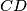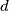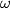### IMO Shortlist 1965 problem 3

Kvaliteta:
Avg: 0.0
Težina:
Avg: 0.0
Given the tetrahedron$ABCD$ whose edges$AB$ and$CD$ have lengths$a$ and$b$ respectively. The distance between the skew lines$AB$ and$CD$ is$d$, and the angle between them is$\omega$. Tetrahedron$ABCD$ is divided into two solids by plane$\epsilon$, parallel to lines$AB$ and$CD$. The ratio of the distances of$\epsilon$ from$AB$ and$CD$ is equal to$k$. Compute the ratio of the volumes of the two solids obtained.
Source: Međunarodna matematička olimpijada, shortlist 1965